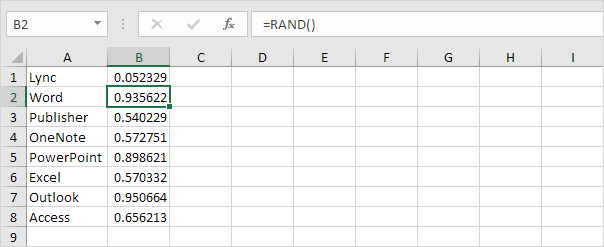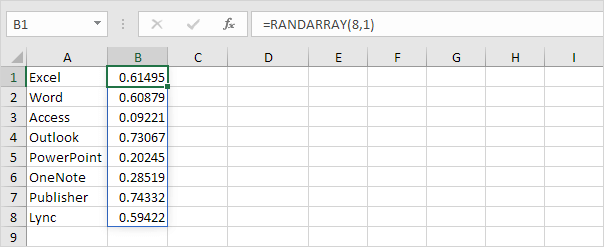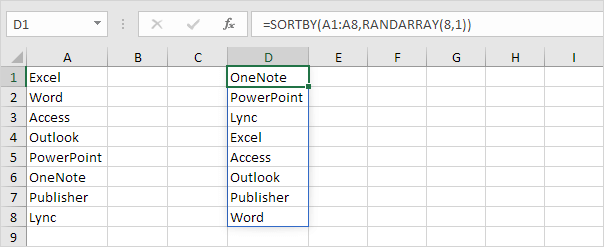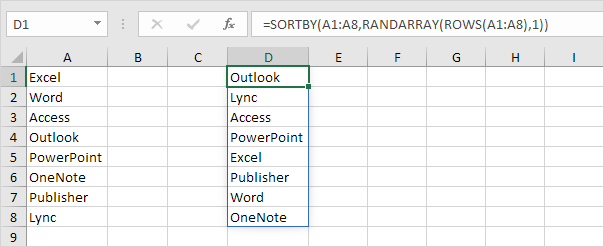# Randomize List

This article teaches you how to randomize (shuffle) a list in Excel. For example, we want to randomize the list in column A below.

1. Select cell B1 and insert the RAND() function.

2. Click on the lower right corner of cell B1 and drag it down to cell B8.3. Click any number in the list in column B.

4. To sort in descending order, on the Data tab, in the Sort & Filter group, click ZA.Result. A random list in column A (sorted on the random numbers above).Note: random numbers change every time a cell on the sheet is calculated. If you don't want this, simply copy the random numbers and paste them as values.

If you have Excel 365 or Excel 2021, use RANDARRAY, SORTBY and ROWS to randomize a list in Excel. The following formula is pretty awesome.

5. First, use the RANDARRAY function to generate a list of random decimal numbers between 0 and 1. The array below consists of 8 rows and 1 column.Note: the RANDARRAY function, entered into cell B1, fills multiple cells. Wow! This behavior in Excel 365/2021 is called spilling.

6. The SORTBY function sorts a range based on the values in a corresponding range. By default, the SORTBY function sorts in ascending order.7. Nest the RANDARRAY function inside the SORTBY function.8. If you have a longer list of say 20 names, change the value 8 to 20 in the formula shown above, or even better, use the ROWS function.Note: the ROWS function simply counts the number of rows in a range.

Go to Next Chapter: Filter